# K Nearest Neighbor : Step by Step Tutorial

In this article, we will cover how K-nearest neighbor (KNN) algorithm works and how to run k-nearest neighbor in R. It is one of the most widely used algorithm for classification problems.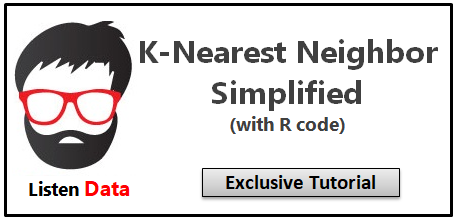K-Nearest Neighbor Simplified

Introduction to K-Nearest Neighbor (KNN)

Knn is a non-parametric supervised learning technique in which we try to classify the data point to a given category with the help of training set. In simple words, it captures information of all training cases and classifies new cases based on a similarity.
Predictions are made for a new instance (x) by searching through the entire training set for the K most similar cases (neighbors) and summarizing the output variable for those K cases. In classification this is the mode (or most common) class value.

How KNN algorithm works

Suppose we have height, weight and T-shirt size of some customers and we need to predict the T-shirt size of a new customer given only height and weight information we have. Data including height, weight and T-shirt size information is shown below -

Height (in cms) Weight (in kgs) T Shirt Size
158 58 M
158 59 M
158 63 M
160 59 M
160 60 M
163 60 M
163 61 M
160 64 L
163 64 L
165 61 L
165 62 L
165 65 L
168 62 L
168 63 L
168 66 L
170 63 L
170 64 L
170 68 L

Step 1 : Calculate Similarity based on distance function

There are many distance functions but Euclidean is the most commonly used measure. It is mainly used when data is continuous. Manhattan distance is also very common for continuous variables.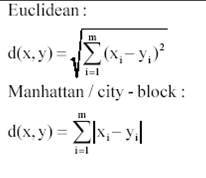Distance Functions

The idea to use distance measure is to find the distance (similarity) between new sample and training cases and then finds the k-closest customers to new customer in terms of height and weight.

New customer named 'Monica' has height 161cm and weight 61kg.

Euclidean distance between first observation and new observation (monica) is as follows -
=SQRT((161-158)^2+(61-58)^2)
Similarly, we will calculate distance of all the training cases with new case and calculates the rank in terms of distance. The smallest distance value will be ranked 1 and considered as nearest neighbor.

Step 2 : Find K-Nearest Neighbors

Let k be 5. Then the algorithm searches for the 5 customers closest to Monica, i.e. most similar to Monica in terms of attributes, and see what categories those 5 customers were in. If 4 of them had ‘Medium T shirt sizes’ and 1 had ‘Large T shirt size’ then your best guess for Monica is ‘Medium T shirt. See the calculation shown in the snapshot below -Calculate KNN manually

In the graph below, binary dependent variable (T-shirt size) is displayed in blue and orange color. 'Medium T-shirt size' is in blue color and 'Large T-shirt size' in orange color. New customer information is exhibited in yellow circle. Four blue highlighted data points and one orange highlighted data point are close to yellow circle. so the prediction for the new case is blue highlighted data point which is Medium T-shirt size.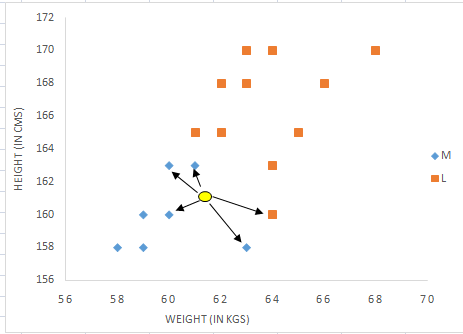KNN: Visual Representation

Assumptions of KNN

1. Standardization

When independent variables in training data are measured in different units, it is important to standardize variables before calculating distance. For example, if one variable is based on height in cms, and the other is based on weight in kgs then height will influence more on the distance calculation. In order to make them comparable we need to standardize them which can be done by any of the following methods :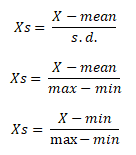Standardization

After standardization, 5th closest value got changed as height was dominating earlier before standardization. Hence, it is important to standardize predictors before running K-nearest neighbor algorithm.Knn after standardization

2. Outlier

Low k-value is sensitive to outliers and a higher K-value is more resilient to outliers as it considers more voters to decide prediction.

Why KNN is non-parametric?

Non-parametric means not making any assumptions on the underlying data distribution. Non-parametric methods do not have fixed numbers of parameters in the model. Similarly in KNN, model parameters actually grows with the training data set - you can imagine each training case as a "parameter" in the model.

KNN vs. K-mean

Many people get confused between these two statistical techniques- K-mean and K-nearest neighbor. See some of the difference below -
1. K-mean is an unsupervised learning technique (no dependent variable) whereas KNN is a supervised learning algorithm (dependent variable exists)
2. K-mean is a clustering technique which tries to split data points into K-clusters such that the points in each cluster tend to be near each other whereas K-nearest neighbor tries to determine the classification of a point, combines the classification of the K nearest points

Can KNN be used for regression?
Yes, K-nearest neighbor can be used for regression. In other words, K-nearest neighbor algorithm can be applied  when dependent variable is continuous. In this case, the predicted value is the average of the values of its k nearest neighbors.

Pros and Cons of KNN

Pros

1. Easy to understand
3. Can be applied to both classification and regression
4. Works easily on multi-class problems

Cons

1. Memory Intensive / Computationally expensive
2. Sensitive to scale of data
3. Not work well on rare event (skewed) target variable
4. Struggle when high number of independent variables
For any given problem, a small value of k will lead to a large variance in predictions. Alternatively, setting  k to a large value may lead to a large model bias.
How to handle categorical variables in KNN?

Create dummy variables out of a categorical variable and include them instead of original categorical variable. Unlike regression, create k dummies instead of (k-1). For example, a categorical variable named "Department" has 5 unique levels / categories. So we will create 5 dummy variables. Each dummy variable has 1 against its department and else 0.

How to find best K value?

Cross-validation is a smart way to find out the optimal K value. It estimates the validation error rate by holding out a subset of the training set from the model building process.

Cross-validation (let's say 10 fold validation) involves randomly dividing the training set into 10 groups, or folds, of approximately equal size. 90% data is used to train the model and remaining 10% to validate it. The misclassification rate is then computed on the 10% validation data. This procedure repeats 10 times. Different group of observations are treated as a validation set each of the 10 times. It results to 10 estimates of the validation error which are then averaged out.

K Nearest Neighbor in R

We are going to use historical data of past win/loss statistics and the corresponding speeches. This dataset comprises of 1524 observations on 14 variables. Dependent variable is win/loss where 1 indicates win and 0 indicates loss. The independent variables are:

1. Proportion of words in the speech showing
a. Optimism
b. Pessimism
c. the use of Past
d. the use of Present
e. the use of Future

2. Number of time he/she mentions his/her own party

3. Number of time he/she mentions his/her opposite parties.

4. Some measure indicating the content of speech showing
a. Openness
b. Conscientiousness
c. Extraversion
d. Agreeableness
e. Neuroticism
f. emotionality

View(data1)

We read the CSV file with the help of read.csv command. Here the first argument is the name of the dataset.  The second argument - Header = TRUE or T implies that the first row in our csv file denotes the headings while header = FALSE or F indicates that the data should be read from the first line and does not involves any headings.
library(caret)
library(e1071)

# Transforming the dependent variable to a factor
data1\$Win.Loss = as.factor(data1\$Win.Loss)
Here we will use caret package in order to run knn. Since my dependent variable is numeric here thus we need to transform it to factor using as.factor().
#Partitioning the data into training and validation data
set.seed(101)
index = createDataPartition(data1\$Win.Loss, p = 0.7, list = F )
train = data1[index,]
validation = data1[-index,]
In order to partition the data into training and validation sets we use createDataPartition() function in caret.

Firstly we set the seed to be 101 so that the same results can be obtained. In the createDataPartition() the first argument is the dependent variable , p denotes how much data we want in the training set; here we take 70% of the data in training set and rest in cross validation set, list = F denotes that the indices we obtain should be in form of a vector.
# Explore data
dim(train)
dim(validation)
names(train)
The dimensions of training and validation sets are checked via dim(). See first 6 rows of training dataset -

```   Win.Loss   Optimism  Pessimism  PastUsed FutureUsed PresentUsed OwnPartyCount
1        X1 0.10450450 0.05045045 0.4381443  0.4948454  0.06701031             2
3        X1 0.11257190 0.04930156 0.4159664  0.5168067  0.06722689             1
5        X1 0.10582640 0.05172414 0.3342618  0.5821727  0.08356546             3
7        X1 0.09838275 0.06401617 0.3240741  0.6018519  0.07407407             6
9        X1 0.10610734 0.04688464 0.3633540  0.5372671  0.09937888             2
10       X1 0.10066128 0.05951506 0.3554817  0.5382060  0.10631229             1
OppPartyCount NumericContent Extra Emoti Agree Consc Openn
1              2    0.001877543 4.041 4.049 3.469 2.450 2.548
3              1    0.002131163 3.463 4.039 3.284 2.159 2.465
5              4    0.002229220 4.658 4.023 3.283 2.415 2.836
7              4    0.002251985 3.727 4.108 3.357 2.128 2.231
9              5    0.002446440 4.119 4.396 3.661 2.572 2.599
10             2    0.002107436 3.800 4.501 3.624 2.117 2.154
```

By default, levels of dependent variable in this dataset is "0" "1". Later when we will do prediction, these levels will be used as variable names for prediction so we need to make it valid variable names.
# Setting levels for both training and validation data
levels(train\$Win.Loss) <- make.names(levels(factor(train\$Win.Loss)))
levels(validation\$Win.Loss) <- make.names(levels(factor(validation\$Win.Loss)))
Here we are using repeated cross validation method using trainControl . Number denotes either the number of folds and ‘repeats’ is for repeated ‘r’ fold cross validation. In this case, 3 separate 10-fold validations are used.
# Setting up train controls
repeats = 3
numbers = 10
tunel = 10

set.seed(1234)
x = trainControl(method = "repeatedcv",
number = numbers,
repeats = repeats,
classProbs = TRUE,
summaryFunction = twoClassSummary)

Using train() function we run our knn; Win.Loss is dependent variable, the full stop after tilde  denotes all the independent variables are there. In ‘data=’ we pass our training set, ‘method=’ denotes which technique we want to deploy, setting preProcess to center and scale tells us that we are standardizing our independent variables

center : subtract mean from values.
scale : divide values by standard deviation.

trControl demands our ‘x’ which was obtained via train( ) and tunelength is always an integer which is used to tune our algorithm.
model1 <- train(Win.Loss~. , data = train, method = "knn",
preProcess = c("center","scale"),
trControl = x,
metric = "ROC",
tuneLength = tunel)

# Summary of model
model1
plot(model1)
```k-Nearest Neighbors

1068 samples
13 predictor
2 classes: 'X0', 'X1'

Pre-processing: centered (13), scaled (13)
Resampling: Cross-Validated (10 fold, repeated 3 times)
Summary of sample sizes: 961, 962, 961, 962, 961, 962, ...
Resampling results across tuning parameters:

k   ROC        Sens       Spec
5  0.8440407  0.6910182  0.8382051
7  0.8537506  0.6847658  0.8520513
9  0.8575183  0.6712350  0.8525796
11  0.8588422  0.6545296  0.8592152
13  0.8585478  0.6560976  0.8556333
15  0.8570397  0.6432249  0.8648329
17  0.8547545  0.6448509  0.8627894
19  0.8520574  0.6336043  0.8632867
21  0.8484632  0.6215447  0.8627894
23  0.8453320  0.6071622  0.8658664

ROC was used to select the optimal model using the largest value.
The final value used for the model was k = 11.
```
Finally to make predictions on our validation set, we use predict function in which the first argument is the formula to be applied and second argument is the new data on which we want the predictions.

# Validation
valid_pred <- predict(model1,validation, type = "prob")

#Storing Model Performance Scores
library(ROCR)
pred_val <-prediction(valid_pred[,2],validation\$Win.Loss)

# Calculating Area under Curve (AUC)
perf_val <- performance(pred_val,"auc")
perf_val

# Plot AUC
perf_val <- performance(pred_val, "tpr", "fpr")
plot(perf_val, col = "green", lwd = 1.5)

#Calculating KS statistics
ks <- max(attr(perf_val, "y.values")[] - (attr(perf_val, "x.values")[]))
ks

The Area under curve (AUC) on validation dataset is 0.8642.

Special thanks to  Ekta Aggarwal for her contribution in this article. She is a co-author of this article. She is a Data Science enthusiast, currently in the final year of her post graduation in statistics from Delhi University.Related PostsDeepanshu founded ListenData with a simple objective - Make analytics easy to understand and follow. He has over 8 years of experience in data science. During his tenure, he has worked with global clients in various domains like Banking, Insurance, Telecom and Human Resource.

6 Responses to "K Nearest Neighbor : Step by Step Tutorial"
1.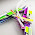Really you explained it where well u deserve my salute u clear my all doubt with best example us president

2.In the R code, what if I wanted to use some other distance method other than Eucledian?

3.Really well explained. Thanks for sharing that. cyberflix tv is a great app that you can use to stream movies on android.

4.Well Explained.

Thank you so much..

5.Hi, I'm really struggling to understand why the standardisation is necessary? If KNN is just a comparison of distances apart, then surely its expected that the variable with the larger range will have more influence on distance, why not just use the distances as they are since it is what it is. In the example, after standardization the 5th closest value changed but thats expected since the numbers have changed so how do you know its more accurate than before, and what exactly does standardisation really do that allows the variables to be more comparable? Sorry for my beginner questions, your tutorials are actually the best I've found after browsing many blogs, books and courses so thanks for this.

1.If you do not standardize your data then the variables in large valued units will dominate the computed distance and variables that are measured in small valued units will contribute very little. It's nothing to do with KNN. Standardisation is also required when you perform clustering because distance function is involved.

Next → ← Prev
Love this Post? Spread the Word!
Share